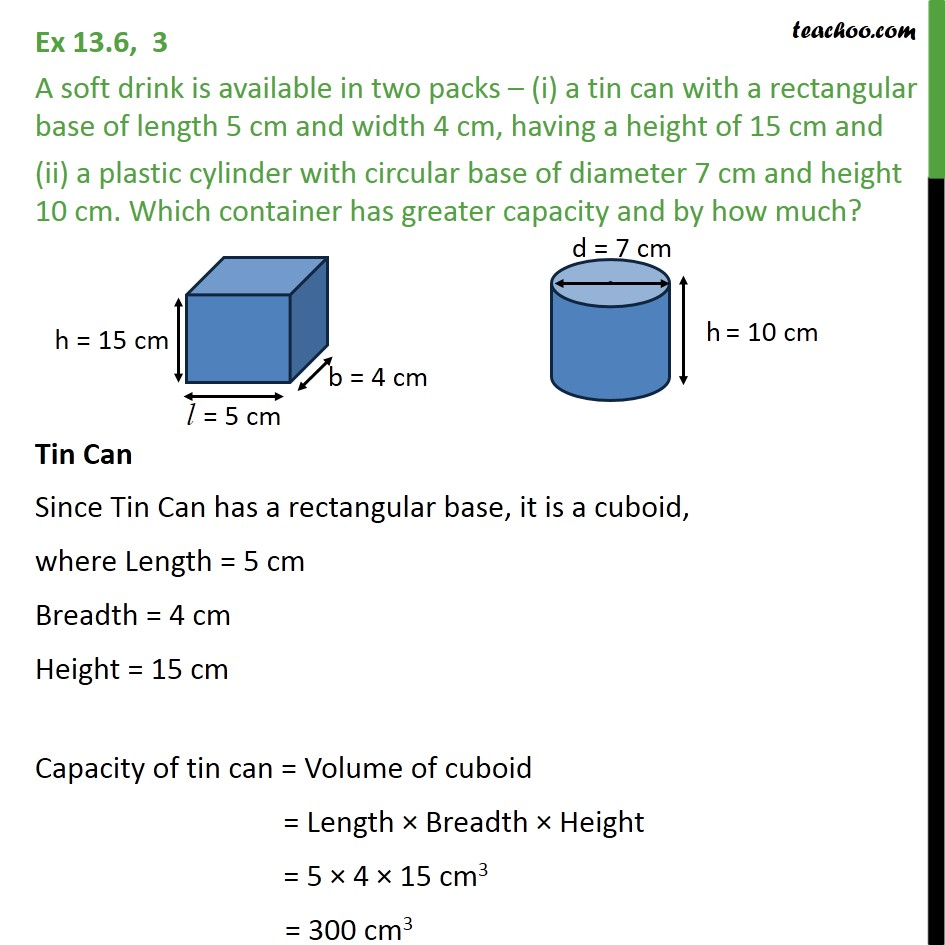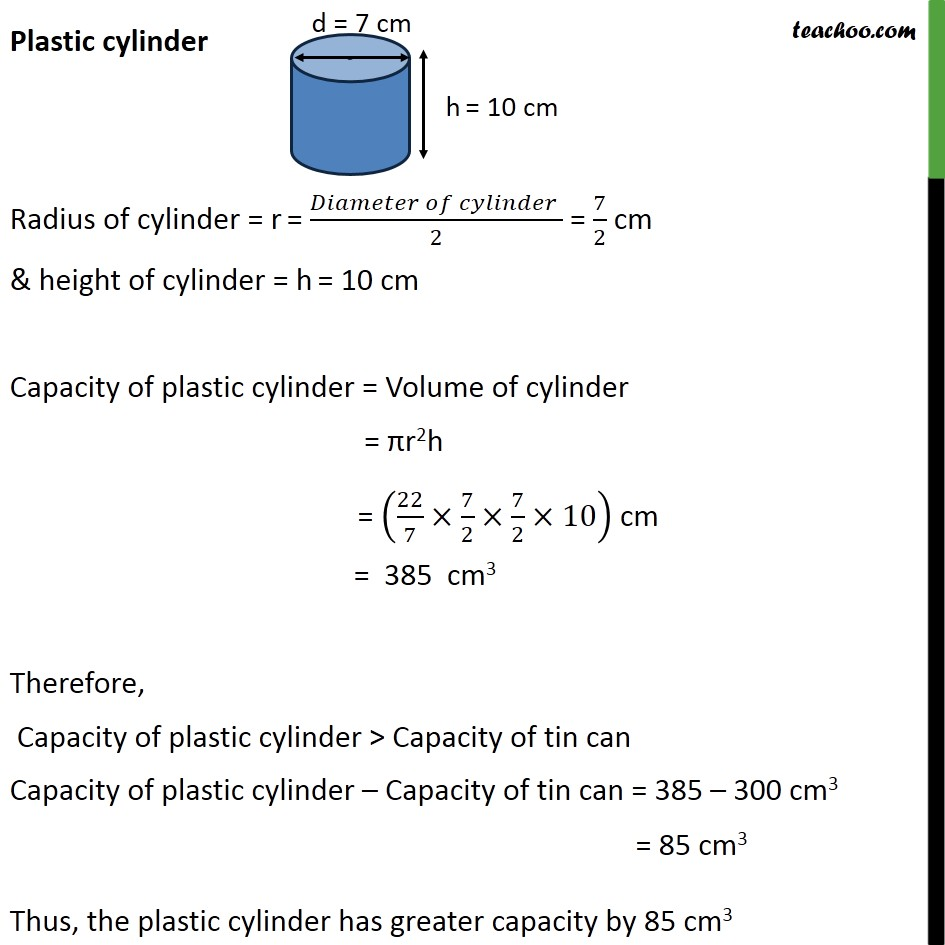Volume of Cylinder

Chapter 11 Class 9 Surface Areas and Volumes
Serial order wiseLearn in your speed, with individual attention - Teachoo Maths 1-on-1 Class

### Transcript

Question 3 A soft drink is available in two packs (i) a tin can with a rectangular base of length 5 cm and width 4 cm, having a height of 15 cm and (ii) a plastic cylinder with circular base of diameter 7 cm and height 10 cm. Which container has greater capacity and by how much? Tin Can Since Tin Can has a rectangular base, it is a cuboid, where Length = 5 cm Breadth = 4 cm Height = 15 cm Capacity of tin can = Volume of cuboid = Length Breadth Height = 5 4 15 cm3 = 300 cm3 Plastic cylinder Radius of cylinder = r = ( )/2 = 7/2 cm & height of cylinder = h = 10 cm Capacity of plastic cylinder = Volume of cylinder = r2h = (22/7 7/2 7/2 10) cm = 385 cm3 Therefore, Capacity of plastic cylinder > Capacity of tin can Capacity of plastic cylinder Capacity of tin can = 385 300 cm3 Thus, the plastic cylinder has greater capacity by 85 cm3